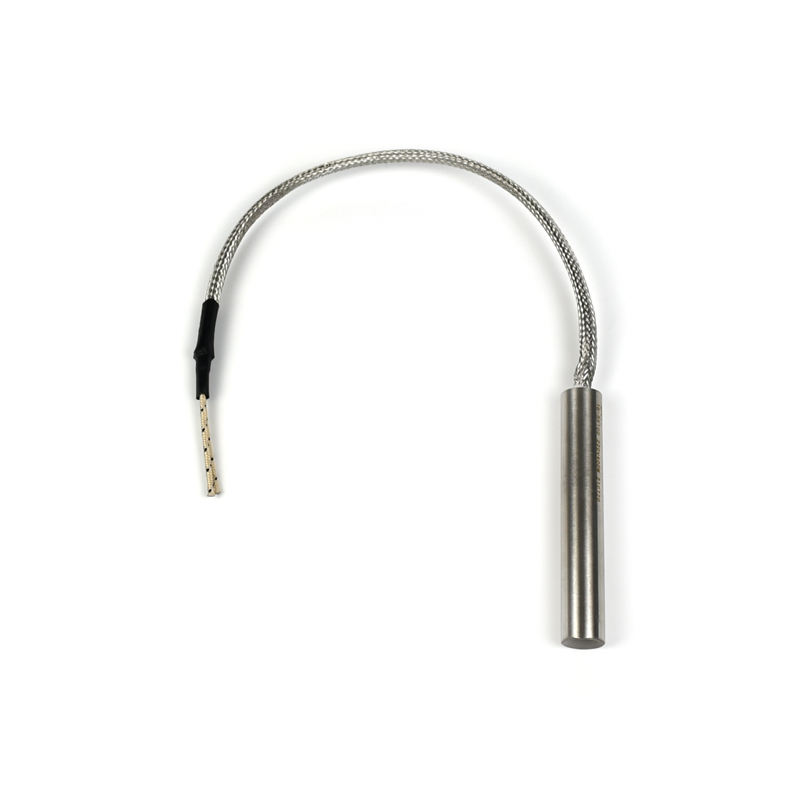Heating Element Manufacturer

How to connect 220V voltage to 380V electric heating tube?

Home    更新    How to connect 220V voltage to 380V electric heating tube?We know that there are 4 commonly used wiring methods for heating pipes:

1. Series connection

When connected in series, the total voltage of the heating tubes is equal to the sum of the voltages of each phase, so the total voltage of the three 220V heating tubes is 220*3=660V. This wiring method is not suitable.

2. Parallel

The total voltage of the heating tubes is equal to the voltage of each phase when connected in parallel, so the total voltage of the three 220V heating tubes is also 220V, obviously it is not possible to connect to a 380V power supply.

3. Delta wiring method

In this connection method, the total voltage of the heating tube is equal to the phase voltage, so the three 220V electric heating tubes should be connected to the 220V power supply voltage.

4. Star (Y) type wiring method

When the star connection is used, the total voltage of the heating tube is equal to the voltage of each phase *1.732, and the three 220V heating tubes should be 220*1.732 approximately equal to 380.

2021年11月6日 10:51